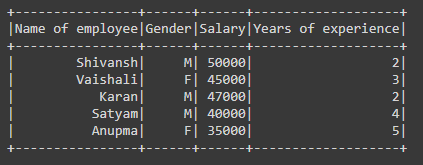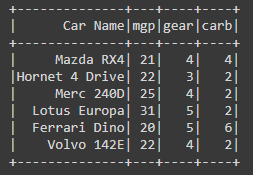# How to create PySpark dataframe with schema ?

• Difficulty Level : Hard
• Last Updated : 09 May, 2021

In this article, we will discuss how to create the dataframe with schema using PySpark. In simple words, the schema is the structure of a dataset or dataframe.

### Functions Used:

For creating the dataframe with schema we are using:

Syntax: spark.createDataframe(data,schema)

Parameter:

• data – list of values on which dataframe is created.
• schema – It’s the structure of dataset or list of column names.

where spark is the SparkSession object.

Example 1:

• In the below code we are creating a new Spark Session object named ‘spark’.
• Then we have created the data values and stored them in the variable named ‘data’ for creating the dataframe.
• Then we have defined the schema for the dataframe and stored it in the variable named as ‘schm’.
• Then we have created the dataframe by using createDataframe() function in which we have passed the data and the schema for the dataframe.
• As dataframe is created for visualizing we used show() function.

## Python

 `# importing necessary libraries``from` `pyspark.sql ``import` `SparkSession`` ` `# function to create new SparkSession``def` `create_session():``  ``spk ``=` `SparkSession.builder \``      ``.master(``"local"``) \``      ``.appName(``"Geek_examples.com"``) \``      ``.getOrCreate()``  ``return` `spk`` ` `# main function``if` `__name__ ``=``=` `"__main__"``:`` ` `  ``# calling function to create SparkSession``  ``spark ``=` `create_session()`` ` `  ``#  creating data for creating dataframe ``  ``data ``=` `[``    ``(``"Shivansh"``,``"M"``,``50000``,``2``),``    ``(``"Vaishali"``,``"F"``,``45000``,``3``),``    ``(``"Karan"``,``"M"``,``47000``,``2``),``    ``(``"Satyam"``,``"M"``,``40000``,``4``),``    ``(``"Anupma"``,``"F"``,``35000``,``5``)``  ``]`` ` `  ``# giving schema``  ``schm``=``[``"Name of employee"``,``"Gender"``,``"Salary"``,``"Years of experience"``]`` ` `  ``# creating dataframe using createDataFrame()``  ``# function in which pass data and schema``  ``df ``=` `spark.createDataFrame(data,schema``=``schm)`` ` `  ``# visualizing the dataframe using show() function``  ``df.show()`

Output:Example 2:

In the below code we are creating the dataframe by passing data and schema in the createDataframe() function directly.

## Python

 `# importing necessary libraries``from` `pyspark.sql ``import` `SparkSession`` ` `# function to create new SparkSession``def` `create_session():``  ``spk ``=` `SparkSession.builder \``      ``.master(``"local"``) \``      ``.appName(``"Geek_examples.com"``) \``      ``.getOrCreate()``  ``return` `spk`` ` `# main function``if` `__name__ ``=``=` `"__main__"``:`` ` `  ``# calling function to create SparkSession``  ``spark ``=` `create_session()`` ` `  ``# creating dataframe using createDataFrame() ``  ``# function in which pass data and schema``  ``df ``=` `spark.createDataFrame([``    ``(``"Mazda RX4"``,``21``,``4``,``4``),``    ``(``"Hornet 4 Drive"``,``22``,``3``,``2``),``    ``(``"Merc 240D"``,``25``,``4``,``2``),``    ``(``"Lotus Europa"``,``31``,``5``,``2``),``    ``(``"Ferrari Dino"``,``20``,``5``,``6``),``    ``(``"Volvo 142E"``,``22``,``4``,``2``)``  ``],[``"Car Name"``,``"mgp"``,``"gear"``,``"carb"``])`` ` `  ``# visualizing the dataframe using show() function``  ``df.show()`

Output:My Personal Notes arrow_drop_up